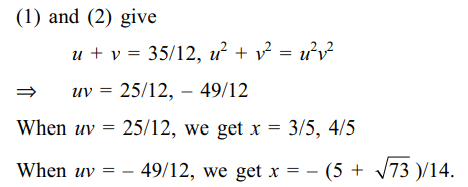1. If three distinct real number a, b and c satisfy $a^{2}\left(a+p\right)=b^{2}\left(b+p\right)=c^{2}\left(c+p\right)$
where $p \epsilon R$ , then value of bc + ca + ab is
a) -p
b) p
c) 0
d) $p^{2}/2$

Explanation: If value of each relation is k, then a, b, c are roots of x3 + px2 – k = 0.

2. The number of integral roots of the equation $x^{4}+\sqrt{x^{4}+20}=22$
is
a) 0
b) 2
c) 4
d) 8

Explanation: Put x4 = y and write3. Let S denote the set of all values of the parameter a for which $x+\sqrt{x^{2}+a}=a$
has no solution, then S equals
a) (– 1, 1)
b) $\left(-\infty ,-1\right)$
c) $\left( -1,\infty\right)$
d) $\left( 0,\infty\right)$

Explanation:4. The number of roots of the equation $\frac{1}{x}+\frac{1}{\sqrt{1-x^{2}}}=\frac{35}{12}$
is
a) 0
b) 1
c) 2
d) 3

Explanation:5. Let S denote the set of all real values of a for which the roots of the equation $x^{2}-2ax+a^{2}-1=0$       lie between 5 and 10, then S equals
a) (– 1, 2)
b) (2, 9)
c) (4, 9)
d) (6, 9)

Explanation:6. Let S denote the set of all values of a for which the roots of the equation
$\left(1+a\right)x^{2}-3ax+4a=0$               (1)
exceed 1, then S equals
a) [– 16/7, 0)
b) (– 16/7, – 1)
c) (– 1, 2)
d) (– 1, 3)

Explanation: Clearly a $\neq$ 0, – 1. Both the roots of (1) will exceed 1 if and only if7. Let S denote the set of all values of S for which the equations
$2x^{2}-2\left(2a+1\right)x+a\left(a+1\right)=0$
has one root less than a and other root greater than a, then S equals
a) (0, 1)
b) (– 1, 0)
c) (0, 1/2)
d) none of these

Explanation:8. Let a, b, p, q $\epsilon$ Q and suppose that
$f \left(x\right) = x^{2}+ax+b=0$     and $g \left(x\right) = x^{3}+px+q=0$
have a common irrational root, then
a) $f \left(x\right)$   divides $g \left(x\right)$
b) $g \left(x\right) \equiv xf \left(x\right)$
c) $g \left(x\right) \equiv \left(x-b-q\right)f\left(x\right)$
d) None of the above

Explanation: Let $\alpha$ $\epsilon$ R – Q be a common root of f(x) =9. The number of irrational solutions of the equation $\sqrt{x^{2}+\sqrt{x^{2}+11}}+\sqrt{x^{2}-\sqrt{x^{2}+11}}=4$               (1)
is
a) 0
b) 2
c) 4
d) 11

Explanation:10. Let S be the set of all non-zero real numbers a such that the quadratic equation $ax^{2}-x+a=0$    has two distinct real roots $x_{1}$ and$x_{2}$ satisfying the inequality $\mid x_{1}-x_{1}\mid < 1$   . Which of the following intervals is(are) a subset(s) of S?
a) $\left(-\frac{1}{2},-\frac{1}{\sqrt{5}}\right)$
b) $\left(-\frac{1}{\sqrt{5}},0\right)$
c) $\left(\frac{1}{\sqrt{5}},\frac{1}{\sqrt{2}}\right)$
d) Both a and c